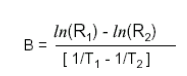# B value of NTC thermistor, The difference between B25/50 and B25/85

NTC thermistors are semiconductor ceramic components made of transition metal oxides,
which have a large negative temperature coefficient. NTC thermistors has a very large negative temperature coefficient.The resistance varies with the ambient temperature or self-heating due to the passage of current. That is, at a certain measurement power, the resistance value decreases rapidly as the temperature rises.Take advantage of this characteristic，The NTC thermistor can be used to detect and control temperature by measuring the resistance value to determine the corresponding temperature.

To understand the difference between B25/50 and B25/85, we have to talk about some general parameters of NTC thermistors.

Zero power resistance value RT

At the specified temperature,
Use causes resistance variation relative to the total measurement error.
A negligible measure of power measured resistance.

Zero Power Resistor R25
Refers to the zero power resistance measured at 25℃.
Unless otherwise noted, it is the design resistance of the thermistor.
It is also the nominal resistance value.

B Value
The B-value is a parameter indicating the sensitivity of the NTC resistance to temperature.
It is defined as the ratio of the difference between the natural logarithm of the zero power resistance value at two temperatures and the difference between the inverse of the two temperatures.
A higher value of B indicates a faster decrease in the resistance of NTC with increasing temperature.
The B-value can be calculated using the following formula:Of which.

RT1 : Zero power resistance at temperature T1 (K).

RT2 : Zero power resistance at temperature T2 (K).

T1, T2 : The two specified temperatures (K).

T1 generally take 25 ℃, when T2 is 50 ℃ is B25/50, when T2 is 85 ℃ is B25/85

B25/50 is the B value calculated from the 25°C resistance value and the 50°C resistance value, and B25/85 is the B value calculated from the 25°C resistance value and the 85°C resistance value.

Generally speaking, B25/85 will be slightly larger than B25/50 for the same thermistor.

# CONCNCT US

Contact: Amy

Phone:

Tel: 金金13510496101

Email: [email protected]

Add: Room2105 , Dongming Building ，Longhua ，Shenzhen, Guangdong, China